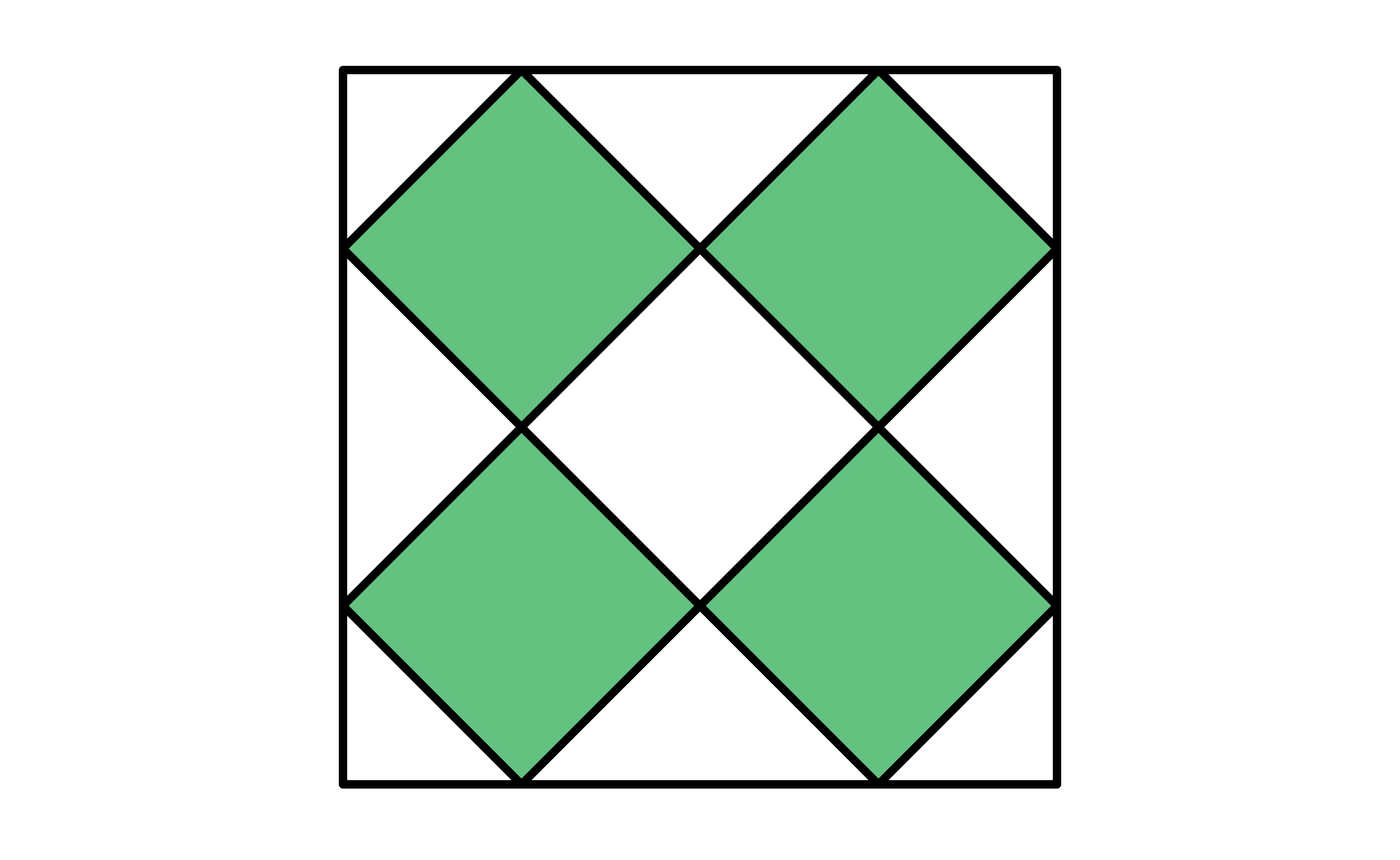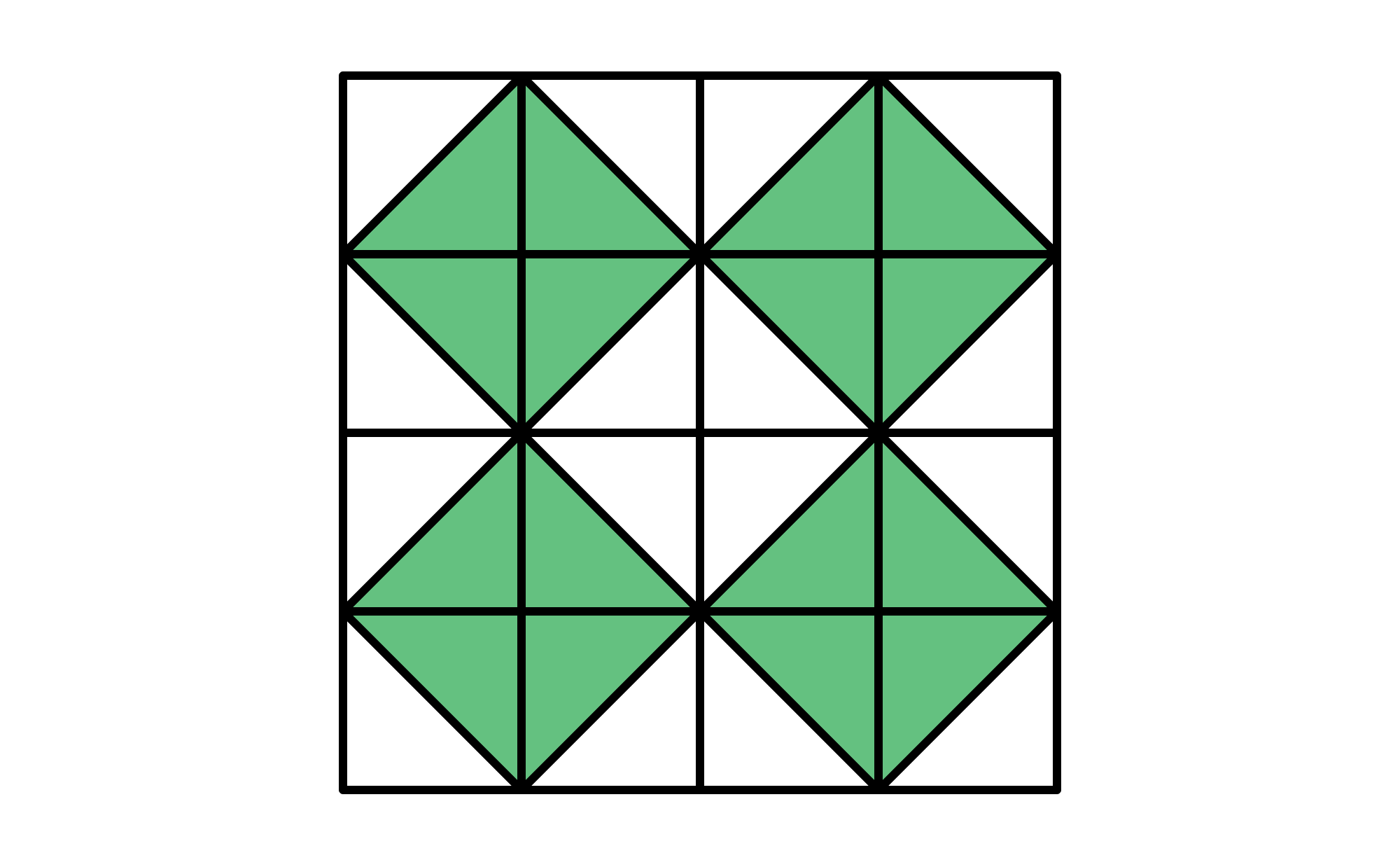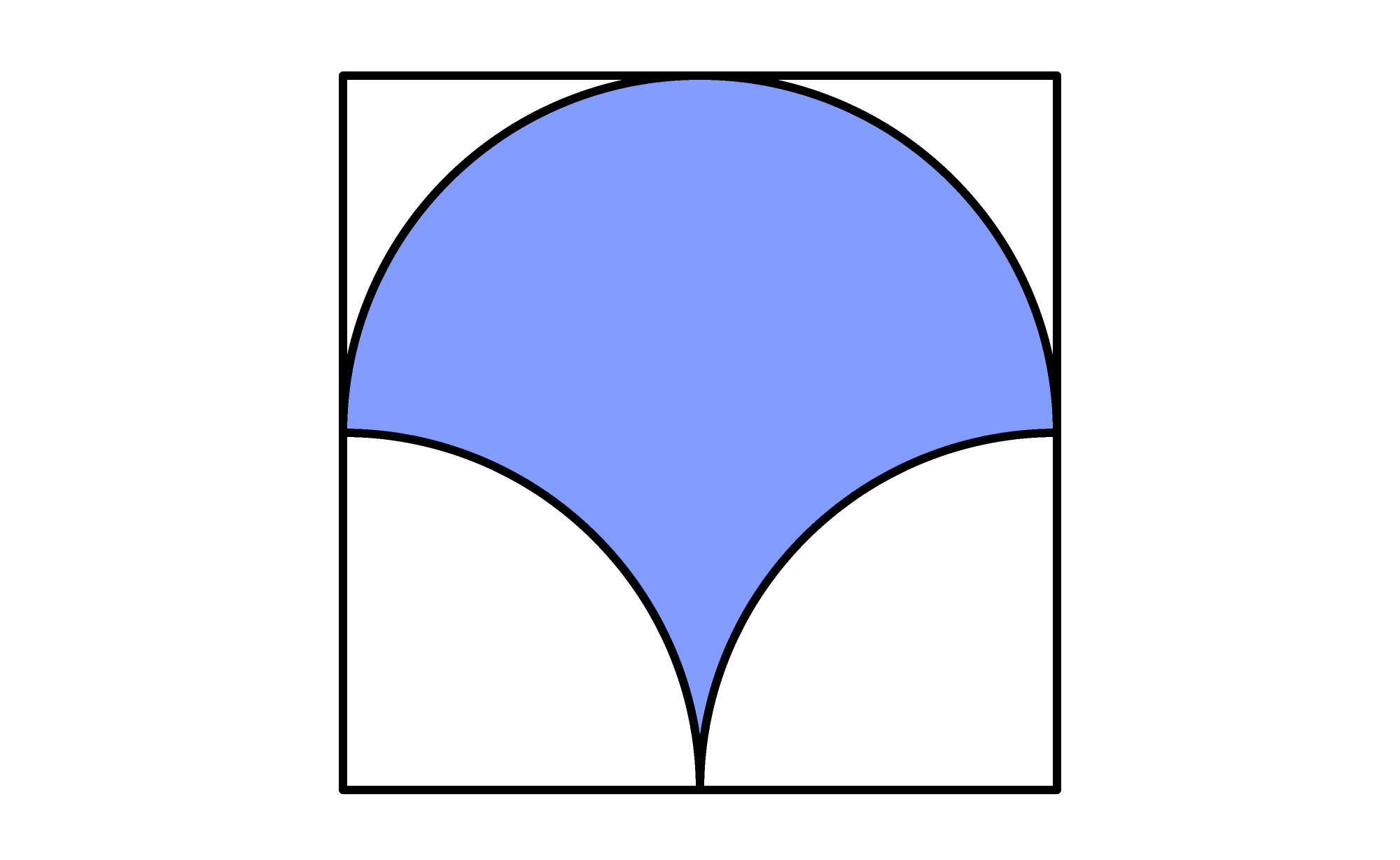Back

## Draw the Line

What fraction of the pattern is colored green?There are four identical green squares, one white square, and some white triangles. We could calculate the areas of these shapes separately and add, or possibly rearrange the triangles to see if we can create white squares. Instead, let's add some lines to the diagram, breaking up the squares and triangles with a grid.For every small green triangle, there is a white triangle with the same area, and vice versa. By adding some lines to the pattern to give it an additional structure, we can see that the area colored green is equal to the area colored white. So, $\frac12$ of the pattern is colored green.

The problem below can also be solved by cutting it into pieces strategically.

# Today's Challenge

The image below is a square containing three circular arcs with the same radius.How does the area of the shaded region compare to the combined area of the unshaded regions?×